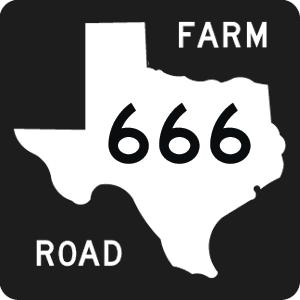CSCE 666: Pattern Analysis Spring 2020 Instructor: Ricardo Gutierrez-Osuna Office: 506A HRBB Phone: 979.845.2942 Email: rgutier@cse.tamu.edu

Course description: Introduction to methods for the analysis, classification and clustering of high-dimensional data in Computer Science applications. Course contents include density and parameter estimation, linear feature extraction, feature subset selection, clustering, Bayesian and geometric classifiers, non-linear dimensionality reduction methods from statistical learning theory and spectral graph theory, Hidden Markov models, and ensemble learning.

Prerequisites: CPSC 206, MATH 222, MATH 411 (or equivalent) and graduate standing in CPSC, CECN, ELEN, CEEN (or permission of the instructor). Basic knowledge of Linear Algebra, Probability and Statistics: algebra of matrices, geometry of Euclidean space, vector spaces and subspaces, basis, linear independence, linear transformations, eigenvalues and eigenvectors, mean, variance, probability and distributions. Programming experience in a high-level language is required.

• Syllabus and tentative schedule: PDF
• MATLAB tutorials:
• CMU’s MATLAB webpage (HTML)
• Project presentation and reporting material:
• Project proposal template (PDF)
• Project presentation grading criteria (PDF)
• Project report grading criteria (PDF)
• Peer review form (PDF)
• Rules for making good presentations, from CMU (HTML)
• How to give a bad talk, by David Patterson (HTML)
• How to prepare your presentations, from Kyoto University (PDF)
• Dazzle'em with style, from OSU (HTML)
• Supplementary material for the lecture notes:
• You and your research, by Richard Hamming (PDF)
• Probability Exercises by John Truxal at SUNY SB (PDF)
• ISOMAP paper by Tenenbaum et al. (PDF)
• LLE paper by Roweis and Saul (PDF)
• Kernel LDA paper by Mika et al. (PDF)
• SVM tutorial paper by Burges (PDF)
• ICA tutorial paper by Hyvarinen and Oja (PDF)
• HMM tutorial by Rabiner (PDF)
Lecture notes

This material is NOT intended to be comprehensive, but rather a SUMMARY of the key concepts covered in the lectures. Consult the syllabus for additional reading material from the textbook, which may be included in the tests.

 Topic Lecture slides Introduction to Pattern Recognition PDF Review of Statistics and Probability PDF Linear Algebra and MATLAB PDF Fourier Analysis PDF Bayesian Decision Theory PDF Quadratic Classifiers PDF Parameter Estimation PDF Kernel Density Estimation PDF Nearest Neighbors PDF Linear Discriminant Functions PDF Cross-validation PDF Principal Components (GIF) PDF Fisher's Linear Discriminants PDF Feature Subset Selection PDF Advanced Dimensionality Reduction PDF Mixture Models and EM (MPEG) PDF Statistical Clustering (MPEG) PDF Independent Components Analysis PDF Support Vector Machines PDF SVMs and Kernel Methods PDF Kernel PCA/LDA PDF Discrete HMMs, Viterbi PDF Baum-Welch and Entropic Training PDF Ensemble Learning PDF

Homework assignments

 Homework # Material 1 hw1.pdf, hw1.zip 2 hw2.pdf, hw2.zip 3 hw3.pdf, hw3.zip